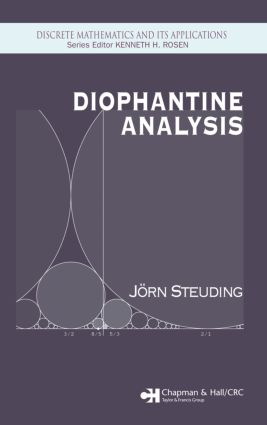# Diophantine Analysis

## 1st Edition

Chapman and Hall/CRC

280 pages | 11 B/W Illus.

##### Purchasing Options:\$ = USD
Hardback: 9781584884828
pub: 2005-05-19
\$115.00
x
eBook (VitalSource) : 9780429129636
pub: 2005-05-19
from \$28.98

FREE Standard Shipping!

### Description

While its roots reach back to the third century, diophantine analysis continues to be an extremely active and powerful area of number theory. Many diophantine problems have simple formulations, they can be extremely difficult to attack, and many open problems and conjectures remain.

Diophantine Analysis examines the theory of diophantine approximations and the theory of diophantine equations, with emphasis on interactions between these subjects. Beginning with the basic principles, the author develops his treatment around the theory of continued fractions and examines the classic theory, including some of its applications. He also explores modern topics rarely addressed in other texts, including the abc conjecture, the polynomial Pell equation, and the irrationality of the zeta function and touches on topics and applications related to discrete mathematics, such as factoring methods for large integers.

Setting the stage for tackling the field's many open problems and conjectures, Diophantine Analysis is an ideal introduction to the fundamentals of this venerable but still dynamic field. A detailed appendix supplies the necessary background material, more than 200 exercises reinforce the concepts, and engaging historical notes bring the subject to life.

### Reviews

"This nicely written textbook provides a down-to-earth introduction to various aspects of Diophantine approximations, transcendence, theory and Diophantine equations….I would not hesitate to recommend this book to anybody wishing to learn something about Diophantine analysis…"

Mathematical Reviews, Issue 2006a

"The book offers a nice introduction to fundamentals of number theory with the emphasis of Diophantine analysis and Diophantine approximation and their mutual interactions. …The reader finds many interesting basic principles and results, which can be used as a springboard to a deeper study of the subject. …The book is written in a lively and lucid style and is principally self-contained and requires only standard mathematical background. It is thus directed not only to graduate or advanced undergraduate students, but to everybody interest in this important part of number theory."

-EMS Newsletter, March 2006

INTRODUCTION: BASIC PRINCIPLES

Who was Diophantus?

Pythagorean triples

Fermat's last theorem

The method of infinite descent

Irrationality of e

Irrationality of pi

Approximating with rationals

Linear diophantine equations

Exercises

CLASSICAL APPROXIMATION THEOREMS

Dirichlet's approximation theorem

A first irrationality criterion

The order of approximation

Kronecker's approximation theorem

Billiard

Uniform distribution

The Farey sequence

Mediants and Ford circles

Hurwitz' theorem

Exercises

CONTINUED FRACTIONS

The Euclidean algorithm revisited and calendars

Finite continued fractions

Interlude: Egyptian fractions

Infinite continued fractions

Approximating with convergents

The law of best approximations

Consecutive convergents

The continued fraction for e

Exercises

THE IRRATIONALITY OF z(3)

The Riemann zeta-function

Apéry's theorem

Approximating z(3)

A recursion formula

The speed of convergence

Final steps in the proof

An irrationality measure

A non-simple continued fraction

Beukers' proof

Notes on recent results

Exercises

Fibonacci numbers and paper folding

Periodic continued fractions

Galois' theorem

Square roots

Equivalent numbers

Serret's theorem

The Marko® spectrum

Notes on the metric theory

Exercises

THE PELL EQUATION

The cattle problem

Lattice points on hyperbolas

An infinitude of solutions

The minimal solution

The group of solutions

The minus equation

The polynomial Pell equation

Nathanson's theorem

Notes for further reading

Exercises

FACTORING WITH CONTINUED FRACTIONS

The RSA cryptosystem

A diophantine attack on RSA

An old idea of Fermat

CFRAC

Examples of failures

Weighted mediants and a refinement

Notes on primality testing

Exercises

GEOMETRY OF NUMBERS

Minkowski's convex body theorem

General lattices

The lattice basis theorem

Sums of squares

Applications to linear and quadratic forms

The shortest lattice vector problem

Gram-Schmidt and consequences

Lattice reduction in higher dimensions

The LLL-algorithm

The small integer problem

Notes on sphere packings

Exercises

TRANSCENDENTAL NUMBERS

Algebraic vs. transcendental

Liouville's theorem

Liouville numbers

The transcendence of e

The transcendence of pi

Squaring the circle?

Notes on transcendental numbers

Exercises

THE THEOREM OF ROTH

Roth's theorem

Thue equations

Finite vs. infinite

Differential operators and indices

Outline of Roth's method

Siegel's lemma

The index theorem

Wronskians and Roth's lemma

Final steps in Roth's proof

Notes for further reading

Exercises

THE ABC-CONJECTURE

Hilbert's tenth problem

The ABC-theorem for polynomials

Fermat's last theorem for polynomials

The polynomial Pell equation revisited

The abc-conjecture

LLL & abc

The ErdÄos-Woods conjecture

Fermat, Catalan & co.

Mordell's conjecture

Notes on abc

Exercises

Non-Archimedean valuations

Ultrametric topology

Ostrowski's theorem

Curious convergence

Characterizing rationals

Completions of the rationals

p-adic numbers as power series

Error-free computing

Notes on the p-adic interpolation of the zeta-function

Exercises

HENSEL'S LEMMA AND APPLICATIONS

Solving equations in p-adic numbers

Hensel's lemma

Units and squares

Roots of unity

Hensel's lemma revisited

Hensel lifting: factoring polynomials

Notes on p-adics: what we leave out

Exercises

THE LOCAL-GLOBAL PRINCIPLE

One for all and all for one

The theorem of Hasse-Minkowski

The theorems of Chevalley and Warning

Applications and limitations

The local Fermat problem

Exercises

APPENDIX: ALGEBRA AND NUMBER THEORY

Groups, rings, and fields

Prime numbers

Riemann's hypothesis

Modular arithmetic

Polynomials

Algebraic number fields

Kummer's work on Fermat's last theorem

BIBLIOGRAPHY

INDEX

### Subject Categories

##### BISAC Subject Codes/Headings:
MAT012000
MATHEMATICS / Geometry / General
MAT022000
MATHEMATICS / Number Theory
MAT036000
MATHEMATICS / Combinatorics